# Probability distribution

In probability and statistics distribution is a characteristic of a random variable, describes the probability of the random variable in each value.

Each distribution has a certain probability density function and probability distribution function.

Though there are indefinite number of probability distributions, there are several common distributions in use.

### Cumulative distribution function

The probability distribution is described by the cumulative distribution function F(x),

which is the probability of random variable X to get value smaller than or equal to x:

F(x) = P(Xx)

#### Continuous distribution

The cumulative distribution function F(x) is calculated by integration of the probability density function f(u) of continuous random variable X.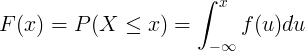#### Discrete distribution

The cumulative distribution function F(x) is calculated by summation of the probability mass function P(u) of discrete random variable X.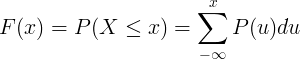### Continuous distributions table

Continuous distribution is the distribution of a continuous random variable.

...

#### Continuous distributions table

Distribution name Distribution symbol Probability density function (pdf) Mean Variance

fX(x)

μ = E(X)

σ2 = Var(X)

X ~ N(μ,σ2)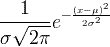μ σ 2
Uniform

X ~ U(a,b)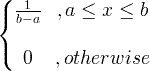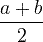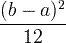Exponential X ~ exp(λ)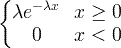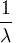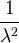Gamma X ~ gamma(c, λ)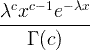x > 0, c > 0, λ > 0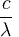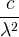Chi square

X ~ χ 2(k)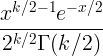k

2k

Wishart
F

X ~ F (k1, k2)

Beta
Weibull
Log-normal

X ~ LN(μ,σ2)

Rayleigh
Cauchy
Dirichlet
Laplace
Levy
Rice
Student's t

### Discrete distributions table

Discrete distribution is the distribution of a discrete random variable.

...

#### Discrete distributions table

Distribution name Distribution symbol Probability mass function (pmf) Mean Variance
fx(k) = P(X=k)

k = 0,1,2,...

E(x) Var(x)
Binomial

X ~ Bin(n,p)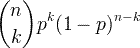np

np(1-p)

Poisson

X ~ Poisson(λ)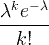λ ≥ 0

λ

λ

Uniform

X ~ U(a,b)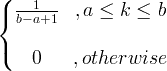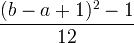Geometric

X ~ Geom(p)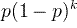Hyper-geometric

X ~ HG(N,K,n)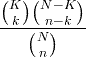N = 0,1,2,...

K = 0,1,..,N

n = 0,1,...,N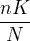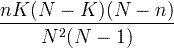Bernoulli

X ~ Bern(p)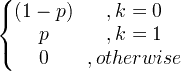p

p(1-p)

Currently, we have around 5612 calculators, conversion tables and usefull online tools and software features for students, teaching and teachers, designers and simply for everyone.